# Linked List in Data Structure

Linked list is a linear data structure. It is a collection of data elements, called nodes pointing to the next node by means of a pointer.

Linked list is used to create trees and graphs.

In linked list, each node consists of its own data and the address of the next node and forms a chain.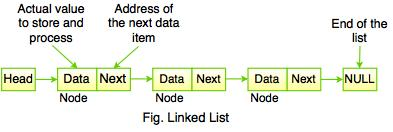The above figure shows the sequence of linked list which contains data items connected together via links. It can be visualized as a chain of nodes, where every node points to the next node.

Linked list contains a link element called first and each link carries a data item. Entry point into the linked list is called the head of the list.

Note: Head is not a separate node but it is a reference to the first node. If the list is empty, the head is a null reference.

Linked list is a dynamic data structure. While accessing a particular item, start at the head and follow the references until you get that data item.

Linked list is used while dealing with an unknown number of objects: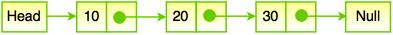In the above diagram, Linked list contains two fields - First field contains value and second field contains a link to the next node. The last node signifies the end of the list that means NULL.

The real life example of Linked List is that of Railway Carriage. It starts from engine and then the coaches follow. Coaches can traverse from one coach to other, if they connected to each other.

• Linked list is dynamic in nature which allocates the memory when required.
• In linked list, stack and queue can be easily executed.
• It reduces the access time.
• Insert and delete operation can be easily implemented in linked list.

• Reverse traversing is difficult in linked list.
• Linked list has to access each node sequentially; no element can be accessed randomly.
• In linked list, the memory is wasted as pointer requires extra memory for storage.

#### Example: Program to create a simple linked list.

#include<stdio.h>
#include <stdlib.h>
int main()
{
struct node
{
int num;
struct node *ptr;
};
typedef struct node NODE;
int count = 0;
int choice = 1;
first = 0;
while(choice)
{
printf("Enter the data item: ");
if(first != 0)
{
}
else
{
}
fflush(stdin);
printf("Do you want to continue(Type 0 or 1)?\n\n");
scanf("%d", &choice);
}
temp->ptr = 0;
temp = first; /* reset temp to the beginning*/
printf("\n Status of the linked list is\n");
while(temp!=0)
{
printf("%d=>", temp->num);
count++;
temp = temp -> ptr;
}
printf("NULL\n");
printf("No. of nodes in the list = %d\n", count);
return 0;
}

Output:A node is declare as below:

Typedef struct node
{
int data;
struct node *next;
}node;

The above code identifies the basic structure of declaring a node. Struct node contains an int data field and a pointer to another node can be defined as struct node* next.

Following are the operations that can be performed on a Linked List:

1. Create
2. Insert
3. Delete
4. Traverse
5. Search
6. Concatenation
7. Display

#### 1. Create

• Create operation is used to create constituent node when required.
• In create operation, memory must be allocated for one node and assigned to head as follows.
Creating first node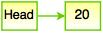#### 2. Insert

• Insert operation is used to insert a new node in the linked list.
• Suppose, we insert a node B(New Node), between A(Left Node) and C(Right Node), it is represented as:
point B.next to B

NewNode.next -> RightNode;

We can insert an element using three cases:

i. At the beginning of the list
ii. At a certain position (Middle)
iii. At the end

Inserting an element

node* nextnode = malloc(sizeof(node));
nextnode -> data = 22;
nextnode -> next = NULL;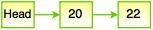The above figure represents the example of create operation, where the next element (i.e 22) is added to the next node by using insert operation.

i. At the beginning of the list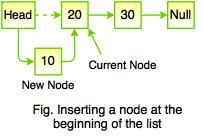ii. At certain position (Middle)While inserting a node in middle of a linked list, it requires to find the current node. The dashed line represents the old node which points to new node.

iii. At the end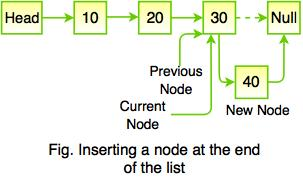While inserting a node at the end of the list, it is achieved by comparing the element values.

#### 3. Delete

• Delete operation is used to delete node from the list.
• This operation is more than one step process.
We can delete an element using three cases:

i. From the beginning of the list
ii. From the middle
iii. From the end

Deleting the element

int delete (node** head, node* n);    // Delete the node n if exists.

i. From the beginning of the list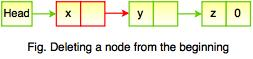When deleting the node from the beginning of the list then there is no relinking of nodes to be performed; it means that the first node has no preceding node. The above figure shows the removing node with x. However, it requires to fix the pointer to the beginning of the list which is shown in the figure below:ii. From the middle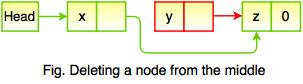Deleting a node from the middle requires the preceding node to skip over the node being removed.

The above figure shows the removal of node with x. It means that there is a need refer to the node before we can remove it.

iii. From the end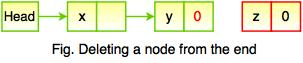Deleting a node from the end requires that the preceding node becomes the new end of the list that points to nothing after it. The above figure shows removing the node with z.

#### 4. Traverse

• Traverse operations is a process of examining all the nodes of linked list from the end to the other end.
• In traverse operation, recursive function is used to traverse a linked list in a reverse order.
The following code snippet represents traversing a node in a linked list:

{
{
}
}

#### 5. Search

• Search operation is used for finding a particular element in a linked list.
• Sequential search is the most common search used on linked list structure.
• Search operation ends with a success if the element is found.
• If the element is not found, search ends in a failure.

#### 6. Concatenation

Concatenation is the process of appending a second list to the end of the first list.

#### 7. Display

• Display operation is used to print each and every node's information.
• This operation displays the complete list.